We improve Knabe’s spectral gap bound for frustration-free translation-invariant local Hamiltonians in 1D. The bound is based on a relationship between global and local gaps. The global gap is the spectral gap of a size-m chain with periodic boundary conditions, while the local gap is that of a subchain of size n < m with open boundary conditions. Knabe proved that if the local gap is larger than the threshold value 1/(n − 1) for some n > 2, then the global gap is lower bounded by a positive constant in the thermodynamic limit m → ∞. Here we improve the threshold to $6n(n+1)$, which is better (smaller) for all n > 3 and which is asymptotically optimal. As a corollary we establish a surprising fact about 1D translation-invariant frustration-free systems that are gapless in the thermodynamic limit: for any such system the spectral gap of a size-n chain with open boundary conditions is upper bounded as O(n−2). This contrasts with gapless frustrated systems where the gap can be Θ(n−1). It also limits the extent to which the area law is violated in these frustration-free systems, since it implies that the half-chain entanglement entropy is $O(1/ϵ)$ as a function of spectral gap ϵ. We extend our results to frustration-free systems on a 2D square lattice.

The spectral gap of a quantum many-body system is the difference between its first excited and ground energies. Here we study the asymptotic scaling of the spectral gap in the thermodynamic limit in which system size diverges. This scaling has physical consequences because the ground state correlation length9,10 and entanglement entropy (at least for one-dimensional systems11,2) are both upper bounded as $Õ(1/ϵ)$ where ϵ is the spectral gap.12 In critical systems the divergence of these quantities is constrained by the rate at which the gap closes. The scaling of the gap can also have algorithmic consequences, since in some cases it determines the time complexity of the quantum adiabatic algorithm.6

The most basic distinction is between gapped and gapless systems.13 How can one determine whether or not a given quantum many-body system is gapped? One might compute the spectral gap for a range of system sizes, and then attempt to extrapolate to thermodynamic limit. Unfortunately, no version of this strategy (or any other strategy) can work in the general case. Indeed, it was recently shown that determining whether or not a translation-invariant two-dimensional system is gapped or gapless is undecidable.5 Even for one-dimensional systems we are not aware of any method.

The gapped versus gapless question seems to be more approachable if one restricts to frustration-free systems. A frustration-free local Hamiltonian, given as a sum $∑iHi$ of local terms, has the property that each of its ground states is also in the ground space of each term Hi. By a constant energy shift and rescaling we may ensure that each term Hi has smallest eigenvalue 0 and $Hi≤1$. In fact, we can (and do) assume without loss of generality that each term Hi is a projector,14 i.e., $Hi2=Hi$. Frustration-freeness means that any ground state $ψ$ of H satisfies $Hiψ=0$ for all i. In the following we specialize to frustration-free translation-invariant 1D systems with nearest-neighbor interactions (later we discuss an extension to 2D systems).

In this setting we are aware of two techniques for bounding the spectral gap in the thermodynamic limit. The Martingale method, due to Nachtergaele,20 establishes a system-size independent lower bound on the spectral gap as long as the ground space satisfies a certain condition (a relationship between the ground space projectors for overlapping contiguous regions of the chain). In this paper we focus on the second technique, due to Knabe,18 which is based on a relationship between the “global” and “local” spectral gaps. The global gap is the spectral gap of a Hamiltonian which describes a size-m chain with periodic boundary conditions. The local gap is the spectral gap of a subchain of size n < m with open boundary conditions. Knabe proved that if the local gap is larger than 1/(n − 1) for some n > 2, then the global gap is lower bounded by a positive constant in the thermodynamic limit m → ∞. This is an easy-to-use criterion for gappedness. In practice one can use exact numerical diagonalization to compute the spectral gap of the open boundary chain for small system sizes n. If for some n one finds a value larger than $1n−1$ then this establishes that the chain with periodic boundary conditions is gapped in the thermodynamic limit.

Our main result is an improvement of the local gap threshold which guarantees a gap in the thermodynamic limit, from 1/(n − 1) to $6n(n+1)$. This improvement is asymptotically optimal as the O(n−2) scaling cannot be improved, see Section II. We also show that the constant 6 is close to optimal, in particular, it cannot be decreased below π2/2 = 4.93.... Since we lower the threshold our bound implies a gap in many cases where Knabe’s does not. On the other hand if one can establish that the spectral gap of a finite-size system exceeds Knabe’s threshold then either result can be used to compute a constant lower bound on the gap in the thermodynamic limit. In that case one may obtain a better (larger) constant using Knabe’s bound.

Our result elucidates a fundamental property of gapless frustration-free one-dimensional systems: roughly, that such systems have spectral gap upper bounded as O(n−2). More precisely, if the system with periodic boundary conditions is gapless in the thermodynamic limit, then the spectral gap of the open boundary chain of size n is at most $6n(n+1)$. This constraint is specific to the frustration-free case; there are many examples of gapless frustrated Hamiltonians which have spectral gap which scales as Θ(n−1). For example, critical systems which have a scaling limit described by a conformal field theory (such as the transverse field Ising model) have spectral gap which scales in this way.

Reference 4 provides a complete classification of translation-invariant frustration-free qubit chains with open boundary conditions, into gapped and gapless cases. Knabe’s result was used to provide an upper bound of 1/(n − 1) on the gap for the gapless cases, see Theorem 1 of Ref. 4. Using our bound in place of Knabe’s in the proof, one obtains a strengthening of that theorem with the improved upper bound $6n(n+1)$.

Knabe described an extension of his bound to two-dimensional systems on a hexagonal lattice, where he obtains a local gap threshold which scales inverse linearly with the diameter of a certain local region18 (he was interested in applying the bound to the AKLT model1). We also extend our results to two dimensions where we find a local gap threshold that scales inverse quadratically with diameter. Here we consider systems with nearest-neighbor interactions on a square lattice. We chose the square lattice because it illustrates a new challenge which arises in two dimensions (see the second paragraph of Section III) and we do not know how to prove a bound for general lattices. For us the global gap is the spectral gap of a system with periodic boundary conditions in both spatial directions, while the local gap is the spectral gap of a smaller-sized region which for technical reasons is chosen to be an n × n patch with two rough and two smooth boundaries (see Figure 1). We prove that if the local gap is greater than 8/n2 for some n then the global gap is lower bounded by a positive constant independent of system size. Note that in two dimensions one could imagine defining a local gap for any connected subgraph of the lattice. It is an open question whether the inverse quadratic (in the diameter) local gap threshold that we obtain for the patch can be generalized to any choice of subgraph.

It is an open question whether or not there is a local gap threshold for translation-invariant systems which may be frustrated. If such a threshold exists in 1D it must be $Ω(1n)$ due to known examples such as the transverse Ising chain. We also note that, to the best of our knowledge, the existence of a local gap threshold for 2D frustrated systems would not contradict the recent undecidability result.5 A local gap threshold would allow one to certify that a system is gapped, but it would not provide an algorithm which decides if a system is gapped or gapless.

Let $ψ$ be the ground state of a local Hamiltonian which describes a 1D chain of n qudits and consider its entanglement entropy S(A) for some contiguous region A ⊆ [n]. The area law11 states that if the system is gapped then S(A) is upper bounded by a constant. Versions of this statement proven in Refs. 11 and 2 also provide an upper bound on the entanglement entropy as a function of the gap ϵn. The strongest known upper bound is2

$S(A)=Õϵn−1.$
(1)

One can ask whether this is tight. In other words what is the maximal violation of the area law in a gapless system? References 8, 16, and 19 construct examples of systems with large entanglement entropy as a function of gap. The strongest known lower bound is given in Ref. 8 which provides an example where $S(A)=Ω̃(ϵn−1/4)$.

Our result directly translates into an upper bound on entanglement entropy as a function of gap for a large class of gapless frustration-free systems. More precisely, consider a translation-invariant frustration-free chain of qudits with periodic boundary conditions which is gapless in the thermodynamic limit. Let ϵn be the spectral gap of the corresponding chain of size n with open boundary conditions. The entanglement entropy S(A) for any ground state $ψ$ and region A ⊆ [n] is trivially upper bounded as

$S(A)≤log(dn)=O1ϵn,$
(2)

where we used our result which states that $ϵn≤6n(n+1)$. We may compare (2) with the area law from Ref. 2 which gives the weaker bound (1) but applies more generally to systems which are gapped in the thermodynamic limit, systems which may be frustrated, and systems without translation invariance.

It is natural to ask if Equation (2) extends to frustration-free systems which are gapped in the thermodynamic limit. If true this would be a stronger version of the area law for such systems. There is an argument in favor of that scenario. It is shown in Ref. 7 that the correlation length ξ in frustration-free systems is upper bounded as

$ξ=O1ϵn,$
(3)

a square-root improvement over the best possible bound for the general (frustrated) case. A non-rigorous physics picture suggests that the entanglement across a cut should be contained within a region around the cut of size O(ξ) where ξ is the correlation length. This suggests that dn in (2) should be replaced by dξ, which along with (3) suggests that (2) should extend to gapped systems. This argument has not been made rigorous, and it was only recently shown that exponential decay of correlations implies an area law at all.3 On the other hand for frustrated systems the same argument suggests the bound S(A) = O(1/ϵn) which was proven (up to a polylog factor) in Ref. 2.

Equations (2) and (3) are both specific to the frustration-free case and represent square-root tightenings of bounds which hold more generally. Is there a unifying explanation for this square-rootiness? At present we do not have a satisfying answer to this question. However it might be interesting to explore the connection between these results and the notion of spectral gap amplification introduced in Ref. 22. That work shows that one can associate a frustrated Hamiltonian H to any frustration-free Hamiltonian H0 such that (a) the ground space of H0 is an (excited) eigenspace of H, and (b) the corresponding eigenvalue gap in the spectrum of H is $ϵ$, where ϵ is the spectral gap of H0.

The rest of this paper is organized as follows. In Section II we state our bound for one-dimensional systems. In Section II A we describe our proof strategy and how it relates to Knabe’s original technique. We prove the bound in Section II B. In Section III we state and prove our bound for two-dimensional systems. The  Appendix contains proofs of two technical lemmas.

In this section we state and prove our spectral gap bound for frustration-free chains. In the following we work in the m-qudit Hilbert space (ℂd)m. Here d is the local qudit dimension which is arbitrary. Consider a one-dimensional chain with periodic boundary conditions described by the Hamiltonian

$Hm∘=∑i=1m−1hi,i+1+hm,1.$

Each term hi,i+1 is a projector (i.e., $hi,i+12=hi,i+1$) which acts nontrivially on two qudits i, i + 1 and as the identity on all other qudits. We assume translation invariance: the action of hi,i+1 on qudits i, i + 1 is described by a d2 × d2 matrix which does not depend on i.

We also define the n-qudit open boundary chain

$Hn=∑i=1n−1hi,i+1.$

We are interested in the case where $Hm∘$ is frustration-free, meaning that it has a nonempty null space. In this case Hn is also frustration-free for all n < m. We write $ϵm∘$ and ϵn for the smallest non-zero eigenvalue of $Hm∘$ and Hn, respectively. Knabe18 proved the following theorem which relates these “global” and “local” spectral gaps.

Theorem 1
Knabe18
Letn > 2 andm > n. Then
$ϵm∘≥n−1n−2ϵn−1n−1.$
Knabe’s theorem directly implies the following corollary, which can be used to establish that the periodic chain $Hm∘$ is gapped in the thermodynamic limit, using information about the gap of a finite size open boundary chain.

Corollary 2
Knabe18

Suppose there exists a positive integern > 2 such that$ϵn>1n−1$. Then there exists a positive constantcsuch that$ϵm∘≥c$for allm.

The contrapositive of this statement is an upper bound on the gap for gapless frustration-free chains: if $Hm∘$ is not gapped in the thermodynamic limit, then $ϵn≤1n−1$ for all n > 2.

Here we improve the local gap threshold $1n−1$ which guarantees a gap in the thermodynamic limit, to $6n(n+1)$.

Theorem 3.
Letn > 2 andm > 2n. Then
$ϵm∘≥56n2+nn2−4ϵn−6n(n+1).$
We describe the proof strategy in Section II A and then we give the proof in Section II B. Before proceeding we give an example that illustrates how tight our bound is. The ferromagnetic Heisenberg model is a qubit chain with nearest-neighbor interaction

$h=1201−1001−10.$

In this example the periodic chain is gapless in the thermodynamic limit. The gap of the open boundary chain of size n is exactly ϵn = 1 − cos(π/n) which is $∼π22n2$ for large n. This shows that the threshold $6n(n+1)$ has the best possible scaling $Θ1n2$, and that, while it might be possible to slightly decrease the constant 6, it cannot be decreased below π2/2 = 4.93....

Suppose we consider the m-qudit chain $Hm∘$ but restrict our attention to a subchain consisting of n qudits {k, k + 1, …, n + k − 1} for some k ∈ [m]. The part of the Hamiltonian which describes this subchain is

$An,k=∑i=kn−2+khi,i+1.$

(Here and throughout this section all indices are to be read modulo m.) It acts on the qudits {k, k + 1, …, n + k − 1} as the open boundary chain Hn and as the identity on all other qudits.

Knabe’s proof of Theorem 118 is based on comparing the operators $(Hm∘)2$ and $∑k=1mAn,k2$. Expanding the squares as sums of operators hi,i+1hj,j+1 one obtains an inequality

$(Hm∘)2+βHm∘≥α∑k=1mAn,k2,$
(4)

where $α=β=1n−2$ are positive coefficients. Since the smallest non-zero eigenvalue of An,k is ϵn, we have $An,k2≥ϵnAn,k$ and therefore

$∑k=1mAn,k2≥ϵn∑k=1mAn,k=ϵnγHm∘,$

where γ = n − 1 is another positive coefficient. Plugging this into (4) and rearranging gives

$(Hm∘)2≥αγϵn−βαγHm∘,$

which establishes that $ϵm∘≥αγϵn−βαγ$. Substituting the values of α, β, γ quoted above one obtains the bound from Theorem 1.

The central difference between our proof and Knabe’s is that we work with deformed versions of the subchain operators An,k. Specifically, let {c0, c1, …, cn−2} be a set of real numbers which satisfies the following three conditions:

$(Positive)cj>00≤j≤n−2,$
(5)
$(Nondecreasing up to midpoint)cj≥cj−11≤j≤n−22,$
(6)
$(Symmetric about midpoint)cj=c(n−2)−j0≤j≤n−22.$
(7)

Define operators

$Bn,k=∑i=kn−2+kci−khi,i+1k∈{1,…,m}.$
(8)

Our proof is based on comparing the operators $(Hm∘)2$ and $∑k=1mBn,k2$. Many of the steps described above can be made to work except that now the coefficients α, β, and γ depend on {c0, c1, …, cn−2}. A suitable choice of the {cj} gives the desired bound.

The idea of deforming the subchain operators in this way was inspired by a calculation due to Alexei Kitaev which establishes an analogous local gap bound for one-dimensional matrices (e.g., tridiagonal matrices).17

One challenge with adapting Knabe’s proof is that while Knabe uses the operator inequality $An,k2≥ϵnAn,k$, the gap of Bn,k is not related to ϵn in a simple way. It is possible to obtain a lower bound

$Bn,k2≥ϵnmin0≤j≤n−2cjBn,k,$

but it turns out that this is not strong enough for our purposes. Happily, we find that the following lemma can be used in place of a stronger lower bound on the eigenvalue gap of Bn,k. The proof of the lemma uses the translation invariance of the periodic chain.

Lemma 4.
Consider the eigenspace of$Hm∘$with eigenvalue$ϵm∘$. There exists a normalized state$ϕ$in this eigenspace which satisfies
$ϕBn,k2ϕ≥ϵn1n−1∑j=0n−2cjϕBn,kϕ,$
(9)
for eachk = 1, …, m.
Proof.
Write T for the space translation operator, that is, the unitary operator which cyclically translates the m qudits by one, i.e.,
$T†hi,i+1T=hi+1,i+2i=1,…,m.$
Since $[T,Hm∘]=0$, we may simultaneously diagonalize T and $Hm∘$. Note that all eigenvalues of T are complex phases e. So we may choose $ϕ$ such that $Hm∘ϕ=ϵm∘ϕ$ and $Tϕ=eiθϕ$ for some θ ∈ ℝ. Using this fact we see that the energy of $ϕ$ is distributed evenly among the terms
$ϕhi,i+1ϕ=ϕT†i−jhj,j+1Ti−jϕ=ϕhj,j+1ϕi,j∈{1,…,m}.$
(10)
Now let Gn,k denote the projector onto the nullspace of Bn,k, and write $Gn,k⊥=I−Gn,k$. If $Gn,k⊥ϕ=0$ then (9) clearly holds since then $Bn,k2ϕ=Bn,kϕ=0$. Next suppose $Gn,k⊥ϕ≠0$ and define
$ϕ̂=1Gn,k⊥ϕGn,k⊥ϕ.$
(11)
Using the fact that $Bn,k=Bn,kGn,k⊥=Gn,k⊥Bn,k$ we get
$ϕBn,k2ϕ=ϕ̂Bn,k2ϕ̂ϕGn,k⊥ϕ$
(12)
$≥ϕ̂Bn,kϕ̂2ϕGn,k⊥ϕ$
(13)
$=ϕ̂Bn,kϕ̂ϕBn,kϕ.$
(14)
Now
$ϕ̂Bn,kϕ̂=∑j=kn−2+kcj−kϕ̂hj,j+1ϕ̂.$
(15)
Using (10) we see that $ϕ̂hi,i+1ϕ̂$ takes the same value for all i ∈ {k, k + 1, …, n − 2 + k}, that is,
$ϕ̂hi,i+1ϕ̂=ϕGn,k⊥hi,i+1Gn,k⊥ϕϕGn,k⊥ϕ=ϕhi,i+1ϕϕGn,k⊥ϕ=ϕhj,j+1ϕϕGn,k⊥ϕ=ϕ̂hj,j+1ϕ̂,$
(16)
for all i, j ∈ {k, …, n − 2 + k}. Using this fact in (15) gives
$ϕ̂Bn,kϕ̂=1n−1∑j=0n−2cjϕ̂∑i=kn−2+khi,i+1ϕ̂≥1n−1∑j=0n−2cjϵn,$
(17)
where in the last inequality we used the fact that $ϕ̂$ is a normalized state orthogonal to the nullspace of the operator $∑i=kn−2+khi,i+1$ and therefore has energy lower bounded by its smallest non-zero eigenvalue, which is ϵn. Plugging (17) into (14) completes the proof.□

Proof.
Let {cj} be a set of numbers which satisfies the conditions (5)-(7), and recall the definition of the deformed subchain operator Bn,k from Equation (8). We begin by taking squares
$(Hm∘)2=Hm∘+∑i=1mhi,i+1hi+1,i+2+hi+1,i+2hi,i+1+∑i,j∈[m]i−j≥2hi,i+1hj,j+1,$
(18)
$Bn,k2=∑i=kn−2+kci−k2hi,i+1+∑i=kn−3+kci−kci+1−khi,i+1hi+1,i+2+hi+1,i+2hi,i+1$
$+∑i,j∈{k,…,n−2+k}i−j≥2ci−kcj−khi,i+1hj,j+1.$
(19)
Next we evaluate $∑k=1mBn,k2$. It will be convenient to define
$d(i,j)=min{i−j,m−i−j}i,j∈[m],$
which is the minimum distance between vertices i and j on the m-cycle. We obtain
$∑k=1mBn,k2=∑j=0n−2cj2Hm∘+∑j=0n−3cjcj+1∑i=1mhi,i+1hi+1,i+2+hi+1,i+2hi,i+1$
$+∑i,j∈{m}n−2≥d(i,j)≥2hi,i+1hj,j+1∑r=0n−2−d(i,j)crcr+d(i,j).$
(20)
It is clear that the first two terms above are obtained by summing the first two terms in (19) over k. In computing the third term we use the hypothesis of the theorem that m > 2n (otherwise one would have to include terms of the form crcr+md(i,j) in (20)).
Now define
$α=∑j=0n−3cjcj+1−1andβ=α∑j=0n−2cj2−∑j=0n−3cjcj+1.$
Using (18) and (20) and the fact that hi,i+1hj,j+1 ≥ 0 whenever d(i, j) ≥ 2 we obtain
$(Hm∘)2−α∑k=1mBn,k2+βHm∘≥∑i,j∈{m}n−2≥d(i,j)≥2hi,i+1hj,j+11−α∑r=0n−2−d(i,j)crcr+d(i,j).$
(21)
We see that the right-hand side is positive semidefinite as long as the coefficients {ci} satisfy
$∑j=0n−3cjcj+1−∑j=0n−x−2cjcj+x≥0x=1,2,…,n−2.$
(22)
However this condition is guaranteed to hold for any choice of the coefficients {cj} which satisfy (5)-(7).
1D Autocorrelation Lemma.
Let {cj : j ∈ {0, 1, …, n − 2}} be any real numbers satisfying conditions (5)-(7). Define
$q(x)=∑j=0n−x−2cjcj+x$
Thenq(x) ≥ q(x + 1) for allx = 0, 1, …, n − 3.
A proof is provided in the  Appendix. Using the lemma we see that the right-hand side of (21) is positive semidefinite and therefore
$(Hm∘)2+βHm∘≥α∑k=1mBn,k2.$
(23)
Now let $ϕ$ be the state whose existence is guaranteed by Lemma 4 and take the expectation value of Equation (23)
$(ϵm∘)2+βϵm∘≥αϕ∑k=1mBn,k2ϕ≥αϵn1n−1∑j=0n−2cjϕ∑k=1mBn,kϕ.$
(24)
Now using the fact that $∑k=1mBn,k=∑j=0n−2cjHm∘$ and the fact that $ϕHm∘ϕ=ϵm∘$, we get
$(ϵm∘)2+βϵm∘≥αn−1∑j=0n−2cj2ϵnϵm∘.$
(25)
Dividing through by $ϵm∘$ and rearranging gives
$ϵm∘≥F(n)ϵn−G(n),$
(26)
where
$F(n)=αn−1∑j=0n−2cj2=1(n−1)∑j=0n−3cjcj+1∑j=0n−2cj2,$
(27)
$G(n)=(n−1)∑j=0n−2cj2−∑j=0n−3cjcj+1∑j=0n−2cj2.$
(28)
We now choose the coefficients {cj} as follows:
$cj=(n−1)+((n−2)j−j2)j=0,…,n−2.$
(29)
It is clear that conditions (5)-(7) are satisfied. To complete the proof we show that this choice gives $F(n)=56n2+nn2−4$ and $G(n)=6n(n+1)$. In fact, one can use Lagrange multipliers to show that the choice (29) is optimal21 in the sense that it minimizes G(n) for all n > 2.
The sums which appear in (27) and (28) can be evaluated exactly,
$∑j=0n−2cj=n3−n6,$
(30)
$∑j=0n−2cj2=n5−n30,$
(31)
$∑j=0n−3cjcj+1=n530−n36+215n.$
(32)
Plugging (30) and (32) into (27) we obtain
$F(n)=1(n−1)(n3−n)2(6/5n5−6n3+7215n)=56(n2+n)(n3−n)n5−5n3+4n=56n2+nn2−4.$
Plugging (30)(32) into (28) gives
$G(n)=(n−1)n3−n6n3−n62=6n(n+1),$
which completes the proof.□

In this section we establish a relationship between local and global gaps for translation-invariant frustration-free systems with nearest-neighbor interactions on a two-dimensional square lattice. Here the global gap is the spectral gap of the system defined on the square lattice with periodic boundary conditions and the local gap is that of a connected subgraph which we refer to as a “patch,” defined below.

Before stating our result we mention an essential difference between the 2D bound given here and the 1D bound from Sec. II. A chain has the property that all pairs of adjacent edges look the same, like. When we square a 1D Hamiltonian $Hm∘$ and write it as a sum of terms hi,i+1hj,j+1 the only ones which are not positive semidefinite are those which come from pairs of adjacent edges, i.e., j = i + 1. In contrast on the 2D square lattice there are two types of pairs of adjacent edges, those which are collinear (e.g.,) and those which are not (e.g.,). Note that for other lattices in two dimensions the number of types of pairs can be different, for example, in the hexagonal lattice which was considered by Knabe18 there is only one type. For the square lattice we are able to make the proof go through in the presence of this additional complication by choosing the shape of the patch in a special way.

Let Λm be an m × m two-dimensional square lattice with periodic boundary conditions in both directions, i.e., a torus. We consider a system of m2 qudits which live at the vertices of Λm. The Hilbert space is (ℂd)m2 where d is the qudit dimension which is arbitrary. We consider a translationally invariant frustration-free Hamiltonian with nearest-neighbor interactions, which we write as

$HmT=∑e∈Λmhe.$

Here the sum is over all edges e in the lattice (here and in the following we do not distinguish between a graph and its edge set). The T superscript is for “torus.” For each edge e there is a projector he which acts nontrivially only on the two qudits at the endpoints of e. In general this projector will not be invariant under swapping these qudits, so we fix an orientation (direction) for each edge e ∈ Λm. On the torus there are two types of edges, horizontal and vertical ones. We assume translation invariance in each direction which means that all horizontal edges are directed the same way (left-to-right, say) and all vertical edges are directed the same way (bottom-to-top). For simplicity we assume that the system is isotropic so that the nontrivial action of he is described by the same d2 × d2 Hermitian matrix, for all edges e. Below we discuss how our bound can be modified very slightly to handle the non-isotropic case where the terms on vertical and horizontal edges may be different. We also assume frustration-freeness which means that the ground energy of $HmT$ is zero.

Ultimately we aim to relate the spectral gap of the Hamiltonian $HmT$ for the torus, and the spectral gap of a Hamiltonian defined on a smaller-sized patch. We choose the shape of the patch in the following way. For each positive even integer n, define a directed n(n + 2)-vertex graph Pn as follows. Start with an undirected grid graph with n rows and n + 2 columns and then remove all the vertical edges in the first and last columns. The result is an n × n square region with two smooth and two rough edges. We direct each horizontal edge left-to-right and each vertical edge bottom-to-top to obtain Pn. It will also be convenient to define another directed graph Qn which is obtained by taking Pn, rotating it by π/2, and then reassigning orientations of the edges so that horizontal edges are directed left-to-right and vertical edges bottom-to-top. The graphs Pn and Qn for the case n = 4 are shown in Figure 1.

We define a patch Hamiltonian which acts on n(n + 2) qudits and has a term for each edge ePn,

$HnP=∑e∈Pnhe.$

We also define $HnQ$ to be the above expression with Pn replaced by Qn. In the isotropic case $HnP$ and $HnQ$ have the same spectrum, and in particular the same spectral gap.

We write $ϵmT$ and $ϵnP$ for the spectral gaps of $HmT$ and $HnP$, respectively. We prove the following 2D analogue of Theorem 3.

Theorem 5.
Letn > 2 be even and letm > 2(n + 2). Then
$ϵmT≥34ϵnP−8n2.$
(33)

We have stated the theorem for the isotropic case where the terms for horizontal and vertical edges are the same, but our proof applies with only cosmetic modification in the non-isotropic case. In that case the gap $ϵnP$ appearing on the right-hand side of (33) should be replaced by $minϵnP,ϵnQ$ where $ϵnQ$ is the spectral gap of $HnQ$.

Since n is even the patch Pn has a center plaquette, shown in Figure 2 for n = 6. For any plaquette k of the torus Λm we define (directed) subgraphs Pn,k and Qn,k of Λm, which are copies of Pn and Qn centered at k. For each of these subgraphs we may consider an associated patch operator which is the sum of all terms in $HmT$ which act on its edges. Below we define deformed versions of these patch operators.

It will be convenient to define a function d(⋅) on the edges of Pn which measures the distance from the center plaquette in the following somewhat unconventional way. The graph Pn contains cocentered subgraphs Pn−2, Pn−4, …, P2; see Figure 2. For any edge ePn we define the distance d(e) from the center plaquette to be the smallest integer $r∈{1,2,…,n2}$ such that e is contained in the cocentered patch P2r. In Figure 2, the edges with d(e) = 1, 2, 3 are shown in blue, red, and black, respectively. We define d(e) for edges eQn in exactly the same way, i.e., with Q replacing P everywhere in the above paragraph.

Let ${c1,c2,…,cn2}$ be a set of positive numbers and define deformed patch operators

$Bn,k=∑e∈Pn,kcd(e)heandCn,k=∑e∈Qn,kcd(e)he.$
(34)

These operators act on the full Hilbert space of m2 qudits, but only act nontrivially on the qudits in the patches Pn,k and Qn,k, respectively. Looking at Equation (34) we see that for each edge there is an operator multiplied by a coefficient which depends only on the distance from the edge to the center plaquette k of the patch.

FIG. 2.

The patch graph P6 contains P4 (in red and blue) and P2 (in blue) as subgraphs which share the same center plaquette, shown in gray. We define a distance function d(e) on the edges e of Pn, which takes integer values between 1 and n/2. In this example the black edges are those with d(e) = 3, the red edges have d(e) = 2, and the blue edges have d(e) = 1.

FIG. 2.

The patch graph P6 contains P4 (in red and blue) and P2 (in blue) as subgraphs which share the same center plaquette, shown in gray. We define a distance function d(e) on the edges e of Pn, which takes integer values between 1 and n/2. In this example the black edges are those with d(e) = 3, the red edges have d(e) = 2, and the blue edges have d(e) = 1.

Close modal

We will choose the coefficients ${c1,c2,…,cn2}$ to satisfy

$(Positive)cj>01≤j≤n2,$
(35)
$(Nonincreasing)cj≤cj−12≤j≤n2.$
(36)

This is the analogue of the constraints (5) and (6) from the 1D case. (Here the analogue of Equation (7) is automatically enforced since, in Equation (34), the coefficient multiplying a given edge depends only on the distance to the center plaquette.)

We now show that the deformed patch operators satisfy a 2D analogue of Lemma 4. Consider the sets of horizontal or vertical edges in the graph Pn and let

be the corresponding averages of horizontal-edge and vertical-edge coefficients (the first equality in each of the above lines is a definition, while the second equality is the result of a simple computation).

Lemma 6.

Proof.
The proof follows that of Lemma 4 very closely. To avoid repetition, we only describe the (small) differences here. On the torus there are two spatial translation generators, unitary operators which shift all qudits by one site in the horizontal and vertical directions, respectively. These operators and the Hamiltonian $HmT$ all mutually commute and we choose $ϕ$ to be a simultaneous eigenvector of all of them. To show that $ϕ$ satisfies (39) one first establishes the analog of (14),
$ϕBn,k2ϕ≥ϕ̂Bn,kϕ̂ϕBn,kϕ$
(41)
(where $ϕ̂$ is defined by (11) with Gn,k the null space of $Bn,k$). Using the fact that $ϕ$ is an eigenstate of both spatial translation operators, one shows (by the same logic as in (16)) that $ϕ̂heϕ̂$ takes the same value for all horizontal edges in Pn,k and likewise for all vertical edges in Pn,k (although the two values may be different). Using this fact we have To go from (43) to (44) we use the fact that $ϕ̂$ is a normalized state orthogonal to the nullspace of the operator $∑e∈Pn,khe$ and therefore has energy lower bounded by its smallest non-zero eigenvalue $ϵnP$. Plugging (44) into (41) gives the desired bound (39). The proof of (40) follows exactly the same steps but with $Cn,k$ instead of $Bn,k$.□

Proof.
We expand the squares
$(HmT)2=∑e∈Λmhe+∑e1,e2∈Λme1≠e2he1he2$
(45)
and
$∑plaquettesk∈ΛmBn,k2+Cn,k2=∑e∈ΛmWn(e,e)he+∑e1,e2∈Λme1≠e2Wn(e1,e2)he1he2.$
(46)
Here Wn(⋅, ⋅ ) is a real-valued function on pairs of edges of Λm, which depends on the coefficients ${c1,c2,…,cn2}$. The value Wn(e1, e2) includes contributions from all terms $Bn,k2$ on the left-hand side of (46) such that e1, e2Pn,k and from all terms $Cn,k2$ such that e1, e2Qn,k. To obtain an explicit expression, define an equivalence relation ∼ on pairs of edges of Λm, which identifies pairs (e1, e2) ∼ (T(e1), T(e2)) that differ only by a lattice translation T. We may then write Wn as a sum of two autocorrelation functions
$Wn(e1,e2)=∑(e,e′)∼(e1,e2)e,e′∈Pn,kcd(e)cd(e′)+∑(e,e′)∼(e1,e2)e,e′∈Qn,kcd(e)cd(e′).$
(47)
The right-hand side does not depend on the choice of center plaquette k used to evaluate it. Note that (47) shows that Wn(⋅, ⋅ ) is invariant if we rotate both of its arguments by π/2. Also note that our assumption that m > 2(n + 2) implies that a subgraph Pn,k or Qn,k extends less than halfway around the torus Λm in each direction. This implies, e.g., that for any pair of edges e, e′ ∈ Pn,k which contribute to the sum in the first term, both the horizontal and vertical distances (number of edges) between them on the torus Λm are the same as those distances within the patch Pn,k (cf. the observation in parentheses after Equation (20)). It will be convenient to introduce a notation for the following function values: We now evaluate these quantities using Equation (47). We have and A straightforward calculation shows that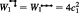and that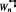satisfies the same recursion (52) as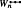. Therefore This equality is not a coincidence—we carefully chose the shape of the patch Pn to make it happen. It is essential for the next step of the proof.
Now define Using Equations (45) and (46) we get
$(HmT)2−α∑plaquettesk∈ΛmBn,k2+Cn,k2+βHmT=∑e1,e2∈Λme1≠e2(1−αWn(e1,e2))he1he2.$
(56)
We now show that each term in the sum on the right-hand side is a positive semidefinite operator. First consider a term where the edges e1 and e2 share a vertex. They may be collinear or not; in either case we have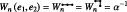and so the corresponding term in (56) vanishes. Next suppose that e1, e2 do not share a vertex. In this case the operators he1 and he2 are commuting projectors and therefore he1he2 ≥ 0. Furthermore, the following lemma states that the coefficient (1 − αWn(e1, e2)) is also non-negative, implying (1 − αWn(e1, e2)) he1he2 ≥ 0.
2D Autocorrelation Lemma.

For any two edgese1, e2 ∈ Λmwhich do not share a vertex, we have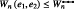.

The proof, given in the  Appendix, uses the representation (47) of Wn(e1, e2) as a sum of two autocorrelation functions and shows that they satisfy an analogue of the monotonicity property stated in the 1D Autocorrelation Lemma.

Thus each term on the right-hand side of (56) is positive semidefinite and therefore
$(HmT)2+βHmT≥α∑plaquettesk∈ΛmBn,k2+Cn,k2.$
Taking the expectation value of this equation in the state $ϕ$ from Lemma 6, applying the lemma, and using the fact that
$∑plaquettesk∈ΛmBn,k+Cn,k=∑e∈Pncd(e)HmT,$
we get Dividing through by $ϵmT$, rearranging, and using (55) gives
$ϵmT≥f(n)ϵnP−g(n),$
(57)
with and Note that
$∑e∈Pncd(e)=∑r=1n/2(16r−8)cr.$
(60)
With this in hand, we now have explicit expressions (as finite sums) for all quantities appearing in Equations (58) and (59), given in Equations (37), (38), (51), (53), and (60). Given a choice of coefficients {c1, c2, …, cn/2} we can evaluate f(n) and g(n) using these expressions. We choose
$cj=n2n2+1−j(j−1)j=1,…,n/2$
(which, as required, is positive and nonincreasing). With this choice all the finite sums can be computed exactly (we omit the details) and we obtain
$f(n)=34n+2n−1n2+23n−43n2+2n−2≥34,$
(61)
$g(n)=n−1n+28n2+23n−43≤8n2.$
(62)
Plugging these bounds into (57) completes the proof.□

We thank Fernando Brandão, Yichen Huang, Alexei Kitaev, Bruno Nachtergaele, Daniel Nagaj, and John Preskill for helpful comments. We also thank Alexei Kitaev for sharing his calculation17 with us. We acknowledge funding provided by the Institute for Quantum Information and Matter, an NSF Physics Frontiers Center (NSF Grant No. PHY-1125565) with support of the Gordon and Betty Moore Foundation (Grant No. GBMF-12500028).

In this appendix we prove the 1D Autocorrelation Lemma and the 2D Autocorrelation Lemma, which establish monotonicity properties of certain autocorrelation functions in one and two dimensions, respectively.

Proof of the 1D Autocorrelation Lemma.
Let {cj : j ∈ {0, 1, …, n − 2}} satisfy (5)-(7). First extend c to a piecewise constant function on the real line as follows:
$c(x)={c⌊x⌋x∈0,n−22,c⌈x⌉x∈n−22,n−2,0x∈(−∞,0)∪(n−2,∞),$
where ⌊x⌋ and ⌈x⌉ indicate, respectively, the largest integer less than or equal to x and the smallest integer larger than x. As an example, consider the choice (with n = 8)
${c0,c1,c2,c3,c4,c5,c6}={1,3,3,4.25,3,3,1}.$
In this example the function c(x) is plotted in red in Figure 3. It is easy to see that any such piecewise constant function can be decomposed as a weighted sum of at most n/2 indicator functions which are each symmetric about the midpoint $n−22$,
$c(x)=∑kfkak(x),$
(A1)
where fk > 0 are positive coefficients and
$ak(x)={1k∈Ik0otherwise.$
Here each Ik ⊆ [0, n − 2] is a closed interval with midpoint $n−22$. In the example considered above we may take I1 = [0, 6], I2 = [1, 5], I3 = [3, 3] and f1 = 1, f2 = 2, f3 = 1.25.
Now using (A1) we obtain an expression for the autocorrelation:
$q(x)=∑j=0n−x−2cjcj+x=∑j=0n−x−2c(j)c(j+x)=∑k,k′fkfk′∑j=0n−x−2ak(j)ak′(j+x).$
To prove the lemma, it suffices to show that, for each pair k, k′, the expression in parentheses is nonincreasing as a function of x ∈ [0, ∞). Using the fact that ak(⋅) is an indicator function associated with the interval Ik we have
$∑j=0n−2−xak(j)ak′(j+x)=Ik∩(Ik′−x),$
(A2)
where Ikx is defined to be the interval Ik shifted to the left by x. To complete the proof note that when x = 0 the intervals Ik and Ikx are cocentered and the size of their intersection is equal to the minimum of their sizes, i.e., $min{Ik,Ik′}$, and that as x increases this intersection can only decrease.□

Next we prove the 2D Autocorrelation Lemma. Here the details are different but the strategy is essentially the same as in the proof given above.

FIG. 3.

We extend {c1, …, cn−2} to a function c(x) on ℝ. Here we show an example with n = 8 and {c0, c1, c2, c3, c4, c5, c6} = {1, 3, 3, 4.25, 3, 3, 1}.

FIG. 3.

We extend {c1, …, cn−2} to a function c(x) on ℝ. Here we show an example with n = 8 and {c0, c1, c2, c3, c4, c5, c6} = {1, 3, 3, 4.25, 3, 3, 1}.

Close modal

Proof of the 2D Autocorrelation Lemma.
Let e1, e2 ∈ Λm be two edges which do not share a vertex. We use the representation (47) of Wn(e1, e2) as a sum of two autocorrelation functions. Denote these two functions, the first and second terms in (47), by A1(e1, e2) and A2(e1, e2). To prove the lemma it is sufficient to show that there exists an edge e3 which shares a vertex with e1 such that
$A1(e1,e2)≤A1(e1,e3)$
(A3)
and
$A2(e1,e2)≤A2(e1,e3).$
(A4)
In particular this shows that Wn(e1, e2) ≤ Wn(e1, e3), where the right-hand side is either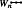or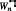(by definition, since e1, e3 share a vertex). The lemma then follows from the fact that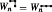(Equation (54)).
Below we provide a proof of (A3); the proof of (A4) is almost identical. We consider
$A1(e1,e2)=∑(e,e′)∼(e1,e2)e,e′∈Pn,kcd(e)cd(e′).$
The right-hand side does not depend on the plaquette k in Λm but for concreteness we imagine fixing some specific choice in the following. This function takes the same value for all pairs of edges in the equivalence class of (e1, e2) (under the relation ∼). Moreover if the function value A1(e1, e2) is non-zero then there are a pair of edges of Pn,k in this equivalence class. For this reason, without loss of generality, below we assume e1, e2 are edges of Pn,k.

It will be helpful to imagine embedding the graph Pn,k in ℝ2, taking each edge to have length 1. Define Te1e2 to be the linear translation of ℝ2 which takes the midpoint of edge e1 into the midpoint of edge e2.15

Let us now fix the edge e3 for which we claim (A3) (and (A4)) holds. We choose e3Pn,k to be the unique edge with the following properties:

1. e3 and e1 share a vertex.

2. e3 and e2 have the same orientation (vertical or horizontal).

3. e3 is closer to e2 than e1 is, i.e., the distance (in ℝ2) between the midpoints of e3 and e2 is smaller than the corresponding distance between the midpoints of e1 and e2.

Let Te1e3 be the translation which maps the midpoint of e1 into the midpoint of e3.

Following the proof of the 1D Autocorrelation Lemma, it will be helpful to decompose the function cd(e) as a weighted sum of indicator functions. Using the fact that c1, c2, …, cn/2 is positive and nonincreasing, we may write
$cd(e)=∑j=1n/2fjaj(e),$
where fj ≥ 0 and
$aj(e)={1d(e)≤j0otherwisee∈Pn,k.$
Then
$A1(e1,e2)=∑j,j′=1n/2fjfj′Rj,j′(e1,e2),$
(A5)
where
$Rj,j′(e1,e2)=∑(e,e′)∼(e1,e2)e,e′∈Pn,kaj(e)aj′(e′).$
We now show that
$Rj,j′(e1,e2)≤Rj,j′(e1,e3),$
(A6)
which along with (A5) implies (A3).
To see why (A6) holds, first note that the function Rj′,j(e1, e2) can be computed as follows. Consider overlapping and cocentered graphs P2j and P2j, both embedded in ℝ2 in the manner described above. Form new graphs $P̃2j$ and $P̃2j′$ by discarding all edges of P2j which are not parallel to e1 and all edges of P2j which are not parallel to e2. Then apply the translation Te1e2 to $P̃2j$. The number of edge midpoints which overlap between $P̃2j′$ and this translated version of $P̃2j$ is equal to the function value Rj,j(e1, e2). Let us write this as
$Rj,j′(e1,e2)=EMP̃2j′,Te1→e2(P̃2j),$
(A7)
where $EM⋅,⋅$ counts the number of edge midpoints which coincide. Likewise we have
$Rj,j′(e1,e3)=EMP̃2j′,Te1→e3(P̃2j).$
(A8)
The translation Te1e2 can be decomposed uniquely as
$Te1→e2=TvThTe1→e3,$
(A9)
where Tv is a vertical translation (i.e., Tv(x) = x + (0, a) for some integer a) and Th is a horizontal translation (Th(x) = x + (b, 0) for integer b). Recall that we chose e3 so that it is closer to e2 than e1 is. Therefore, if it is nontrivial, the translation Th (respectively, Tv) increases the difference between the horizontal (respectively, vertical) coordinates of the center plaquettes of the two graphs. As a result it is not hard to see that each of these translations can only decrease the number of edge midpoints which overlap, i.e.,
$EMP̃2j′,Te1→e3(P̃2j)≥EMP̃2j′,ThTe1→e3(P̃2j)≥EMP̃2j′,TvThTe1→e3(P̃2j).$
(A10)

It may help to refer to Figure 4 which illustrates an example. Equation (A6) then follows directly from (A7)(A10).□

FIG. 4.

Here we take n = 3, j = 2, and j′ = 3 and we illustrate how to compute Rj,j(e1, e2), where the edges e1, e2, and e3 are shown in (a). We start with overlapping cocentered copies of P2j (red in (a)) and P2j (red and black in (a)). Although these are directed graphs we have not drawn the edge orientations since they are irrelevant here. Form new graphs $P̃2j$ and $P̃2j′$, shown in (b), by retaining only the edges which are parallel to e1 and e2, respectively. Then apply the shift Te1e2 to $P̃2j$ (shown in (e)); then Rj,j(e1, e2) = 8 is the number of edge midpoints which overlap between this graph and $P̃2j′$. We can decompose the shift as Te1e2 = TvThTe1e3 where Tv and Th are vertical and horizontal translations, respectively. Subfigures (c) and (d) show $P̃2j′$ along with $Te1→e3(P̃2j)$ and $ThTe1→e3(P̃2j)$, respectively.

FIG. 4.

Here we take n = 3, j = 2, and j′ = 3 and we illustrate how to compute Rj,j(e1, e2), where the edges e1, e2, and e3 are shown in (a). We start with overlapping cocentered copies of P2j (red in (a)) and P2j (red and black in (a)). Although these are directed graphs we have not drawn the edge orientations since they are irrelevant here. Form new graphs $P̃2j$ and $P̃2j′$, shown in (b), by retaining only the edges which are parallel to e1 and e2, respectively. Then apply the shift Te1e2 to $P̃2j$ (shown in (e)); then Rj,j(e1, e2) = 8 is the number of edge midpoints which overlap between this graph and $P̃2j′$. We can decompose the shift as Te1e2 = TvThTe1e3 where Tv and Th are vertical and horizontal translations, respectively. Subfigures (c) and (d) show $P̃2j′$ along with $Te1→e3(P̃2j)$ and $ThTe1→e3(P̃2j)$, respectively.

Close modal
1.
Affleck
,
I.
,
Kennedy
,
T.
,
Lieb
,
E. H.
, and
Tasaki
,
H.
, “
Rigorous results on valence-bond ground states in antiferromagnets
,”
Phys. Rev. Lett.
59
,
799
802
(
1987
).
2.
,
I.
,
Kitaev
,
A.
,
Landau
,
Z.
, and
Vazirani
,
U.
, “
An area law and sub-exponential algorithm for 1D systems
,” e-print arXiv:1301.1162 (
2013
).
3.
Brandão
,
F. G. S. L.
and
Horodecki
,
M.
, “
Exponential decay of correlations implies area law
,”
Commun. Math. Phys.
333
,
761
798
(
2015
).
4.
Bravyi
,
S.
and
Gosset
,
D.
, “
Gapped and gapless phases of frustration-free spin-$12$ chains
,”
J. Math. Phys.
56
(
6
),
061902
(
2015
).
5.
Cubitt
,
T.
,
Garcia-Perez
,
D.
, and
Wolf
,
M.
, “
Undecidability of the spectral gap
,”
Nature
528
,
207
211
(
2015
); e-print arXiv:1502.04573 (
2015
).
6.
Farhi
,
E.
,
Goldstone
,
J.
,
Gutmann
,
S.
, and
Sipser
,
M.
, “
,” e-print arXiv:quant-ph/0001106 (
2000
).
7.
Gosset
,
D.
and
Huang
,
Y.
, “
Correlation length versus gap in frustration-free systems
,”
Phys. Rev. Lett.
116
,
097202
(
2016
); e-print arXiv:1509.06360 (
2015
).
8.
Gottesman
,
D.
and
Hastings
,
M. B.
, “
Entanglement versus gap for one-dimensional spin systems
,”
New J. Phys.
12
(
2
),
025002
(
2010
).
9.
Hastings
,
M. B.
, “
Lieb-Schultz-Mattis in higher dimensions
,”
Phys. Rev. B
69
(
10
),
104431
(
2004
).
10.
Hastings
,
M. B.
, “
Locality in quantum and Markov dynamics on lattices and networks
,”
Phys. Rev. Lett.
93
(
14
),
140402
(
2004
).
11.
Hastings
,
M. B.
, “
An area law for one-dimensional quantum systems
,”
J. Stat. Mech.: Theory Exp.
08
,
P08024
(
2007
).
12.

Here the $Õ(⋅)$ notation hides a polylogarithmic function of $1ϵ$ which is present in the entanglement entropy bound from Ref. 2.

13.

Here we consider a quantum many-body system described by a sequence of Hamiltonians {Hn} indexed by system size n. The system is gapped if the spectral gap of Hn is lower bounded by a positive constant independent of n; otherwise it is gapless.

14.

If Hi is not a projector, we may replace it with the projector Πi orthogonal to its null space. It is not hard to see that the new Hamiltonian $H′=∑iΠi$ has the same zero energy ground space as H and that the spectral gaps ϵ and ϵ′ of these Hamiltonians satisfy ′ ≤ ϵϵ′, where a lower bounds the smallest non zero eigenvalue of each term Hi.

15.

In other words, for x ∈ ℝ2 we have Te1e2(x) = x + e2e1 (here e2, e1 are identified with the coordinates of their midpoints).

16.
Irani
,
S.
, “
Ground state entanglement in one-dimensional translationally invariant quantum systems
,”
J. Math. Phys.
51
(
2
),
022101
(
2010
).
17.
Kitaev
,
A.
, private communication (2015).
18.
Knabe
,
S.
, “
Energy gaps and elementary excitations for certain VBS-quantum antiferromagnets
,”
J. Stat. Phys.
52
(
3-4
),
627
638
(
1988
).
19.
Movassagh
,
R.
and
Shor
,
P. W.
, “
Power law violation of the area law in quantum spin chains
,” e-print arXiv:1408.1657 (
2014
).
20.
Nachtergaele
,
B.
, “
The spectral gap for some spin chains with discrete symmetry breaking
,”
Commun. Math. Phys.
175
(
3
),
565
606
(
1996
).
21.

Note that G(n) is invariant under a rescaling cjacj, so we are free to choose the normalization so that $∑j=0n−2cj=1$. To find the optimum of G(n) we minimize the numerator of (28) subject to this constraint. The solution is equal to (29) (up to an irrelevant rescaling).

22.
Somma
,
R. D.
and
Boixo
,
S.
, “
Spectral gap amplification
,”
SIAM J. Comput.
42
(
2
),
593
610
(
2013
).